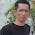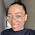## Tuesday, July 6, 2010

### Methods of adjusting ACCUMULATED DEPRECIATION at the date of REVALUATION

When an item of Property, Plant and Equipment is REVALUED, any accumulated depreciation at the date of the revaluation is treated in one of the following ways : (1) Restate accumulated depreciation proportionately with the change in the gross carrying amount of the asset (so that the carrying amount of the asset after revaluation equals its revalued amount); or (2) Eliminate the accumulated depreciation against the gross carrying amount of the asset (IAS 16 par. 35).

Example, Konin Corporation (KC) own buildings with a cost of USD200,000 and estimated useful life of five years. Accordingly, depreciation of USD40,000 per year is anticipated. After two years, KC obtains market information suggesting that a current fair value of the buildings is USD 300,000 and decided to write the building up to a fair value of USD300,000. There are two approaches to apply the revaluation model in IAS 38; the asset and accumulated depreciation can be “grossed up” to reflect the new fair value information, or the asset can be restated on a “net” basis. These two approaches are illustrated below. For both illustrations, the net carrying amount (book value or depreciated cost) immediately prior to the revaluation is USD120,000 (USD 200,000 - (2XUSD40,000)). The net upward revaluation is given by the difference between the fair value and net carrying value, or USD300,000 – USD120,000 = USD180,000.

Option 1. Applying the “gross up” approach, since the fair value after two years of the five-year useful life have already elapsed is found to be USD300,000, the gross fair value (gross carrying amount) must be 5/3 X USD300,000 = USD500,000. In order to have the net carrying value equal to the fair value after two years, the balance in accumulated depreciation needs to be USD200,000. Consequently, the buildings and accumulated depreciation accounts need to be restated upward as follows : buildings up USD300,000 (USD500,000 – USD200,000) and accumulated depreciation USD120,000 (USD200,000 – USD80,000). Alternatively, this revaluation could be accomplished by restating the buildings account and the accumulated depreciation account so that the ratio of net carrying amount to gross carrying amount is 60% (USD120,000/USD200.000) and the net carrying amount is USD300,000. New gross carrying amount is calculated USD300,000/60%=USD500,000.

The following journal entry illustrate the restatement of the accounts :

 Buildings 300,000 Accumulated Depreciation 120,000 Other comprehensive income – gain on revaluation 180,000

And the following table illustrate the restatement of the accounts :

 Original cost Revaluation Total % Gross carrying amount USD200,000 + USD300,000 = USD500,000 100 Accumulated Depreciation 80,000 + 120,000 = 200,000 40 Net carrying amount USD120,000 + USD180,000 = USD300,000 60

After the revaluation, the carrying value of the building is USD300,000 (=USD500,000 – 200,000) and the ratio of net carrying amount to gross carrying amount is 60% (=USD300,000/USD500,000).

This method is often used when an asset is revalued by means of applying an index to determine its depreciated replacement cost.

Option 2. Applying the “netting” approach, KC would eliminate accumulated depreciation of USD80,000 and then increase the building account by USD180,000 so the net carrying amount is USD300,000 (=USD200,000 – USD80,000 + USD180,000). The journal entry as follow :

 Acc. Depreciation 80,000 Buildings 80,000 Buildings 180,000 Other comprehensive income – gain on revaluation 180,000

This method is often used for buildings. In terms of total assets reported in the statement of financial position, option 2 has exactly the same effect as option 1.

However, many users of financial statements, including credit grantors and prospective investors, pay heed to the ratio of net property and equipment as a fraction of the related gross amounts. This is done to assess the relative age of the entity’s productive assets and, indirectly, to estimate the timing and amounts of cash needed for asset replacements. There is a significant diminution of information under the second method. Accordingly, the first approach described above, preserving the relationship between gross and net asset amounts after the revaluation, is recommended as the preferable alternative is the goal is meaningful financial reporting.

Source : WILEY – 2010 Interpretation and Application of IFRS, Barry J. Epstein and Eva K. Jermakowicz

#### 8 comments:

1.Is this updated with the current standards?

2.Yes, as far as I know, the above presentation already in line with current IFRS

3.Thank you so much for clearing this up for me

4.This is really helpful, thank you so much. doubts cleared.

5.good explnation

6.Great. But one little error, you mentioned IAS 38 which is for Intangible Assets.

7.Thank you

8.Please, could you help with two revaluations of an asset in a period? Which periods and values I should take?